# 使用盲反卷积算法对图像进行去模糊

### 步骤 1：读取图像

```I = imread("cameraman.tif"); figure;imshow(I);title("Original Image"); text(size(I,2),size(I,1)+15, ... "Image courtesy of Massachusetts Institute of Technology", ... "FontSize",7,"HorizontalAlignment","right");```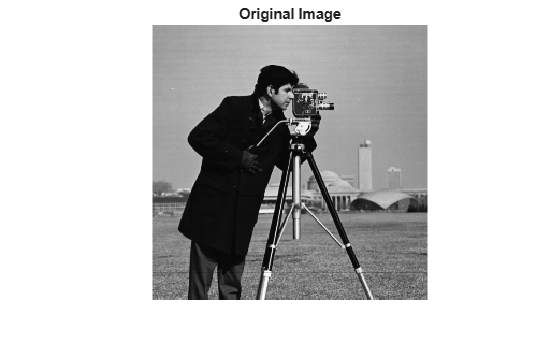### 步骤 2：仿真模糊效果

```PSF = fspecial("gaussian",7,10); Blurred = imfilter(I,PSF,"symmetric","conv"); imshow(Blurred) title("Blurred Image")```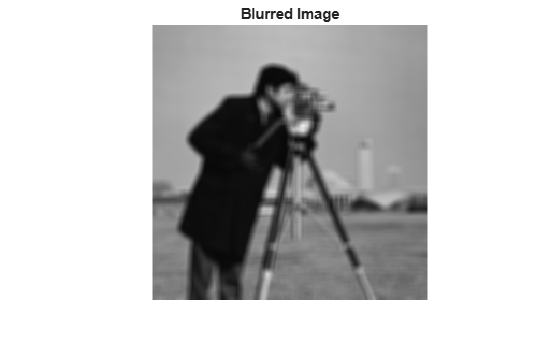### 步骤 3：使用不同大小的 PSF 还原模糊图像

```UNDERPSF = ones(size(PSF)-4); [J1,P1] = deconvblind(Blurred,UNDERPSF); imshow(J1) title("Deblurring with Undersized PSF")```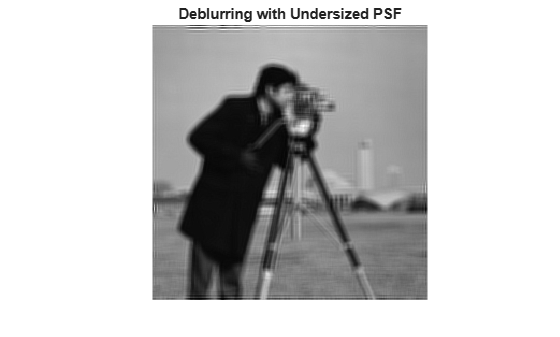```OVERPSF = padarray(UNDERPSF,[4 4],"replicate","both"); [J2,P2] = deconvblind(Blurred,OVERPSF); imshow(J2) title("Deblurring with Oversized PSF")```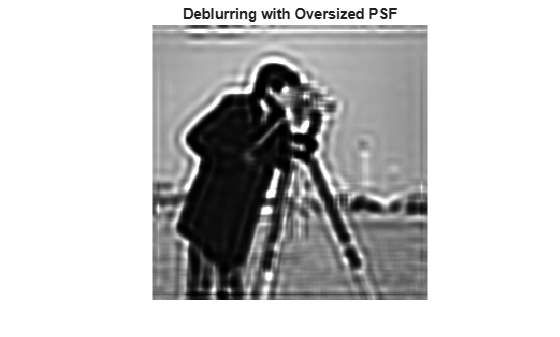```INITPSF = padarray(UNDERPSF,[2 2],"replicate","both"); [J3,P3] = deconvblind(Blurred,INITPSF); imshow(J3) title("Deblurring with INITPSF")```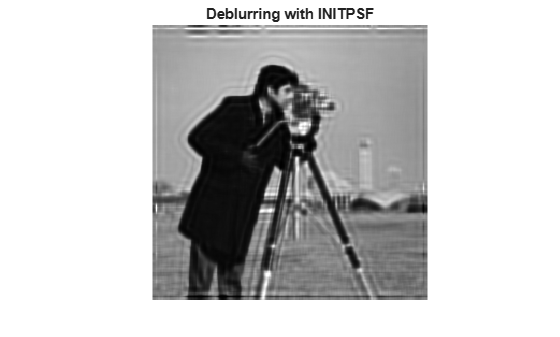### 步骤 4：分析还原的 PSF

```figure; subplot(2,2,1) imshow(PSF,[],"InitialMagnification","fit") title("True PSF") subplot(222) imshow(P1,[],"InitialMagnification","fit") title("Reconstructed Undersized PSF") subplot(2,2,3) imshow(P2,[],"InitialMagnification","fit") title("Reconstructed Oversized PSF") subplot(2,2,4) imshow(P3,[],"InitialMagnification","fit") title("Reconstructed true PSF")```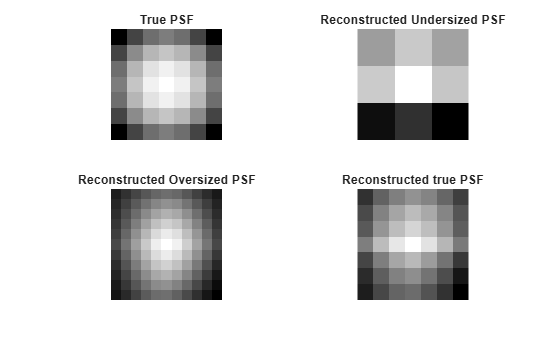### 步骤 5：改进还原

`WEIGHT = edge(Blurred,"sobel",.08);`

```se = strel("disk",2); WEIGHT = 1-double(imdilate(WEIGHT,se));```

```WEIGHT([1:3 end-(0:2)],:) = 0; WEIGHT(:,[1:3 end-(0:2)]) = 0; figure imshow(WEIGHT) title("Weight Array")``````[J,P] = deconvblind(Blurred,INITPSF,30,[],WEIGHT); imshow(J) title("Deblurred Image")```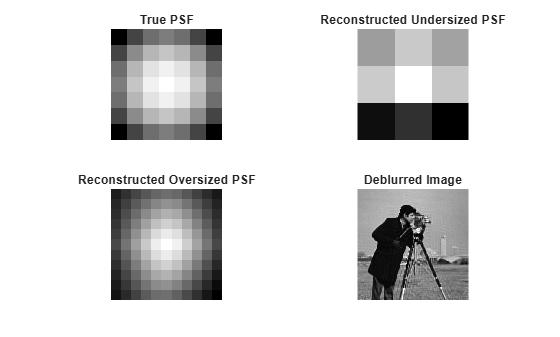### 步骤 6：对 PSF 还原使用附加约束

```P1 = 2; P2 = 2; FUN = @(PSF) padarray(PSF(P1+1:end-P1,P2+1:end-P2),[P1 P2]);```

```[JF,PF] = deconvblind(Blurred,OVERPSF,30,[],WEIGHT,FUN); imshow(JF) title("Deblurred Image")```